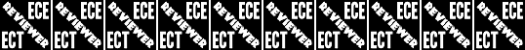# Geas

10 Questions | Total Attempts: 8280SettingsPriority Level: High (ECE)

Related Topics
• 1.
An object which weighs 10 N is dropped from rest from a height of 4 meters above the ground. When it has free-fallen 1 meter its total mechanical energy with respect to the ground is ____.
• A.

2.5 J

• B.

10 J

• C.

30 J

• D.

40 J

• 2.
Which of the following statements about vectors and scalars is TRUE?
• A.

A vector is a large quantity and a scalar is a small quantity

• B.

A scalar quantity has a magnitude and a vector quantity does not

• C.

A vector quantity is described with a direction and a scalar is not

• D.

A scalar quantity depends only upon the initial and final values of the quantity; this is not the case for vector quantities

• 3.
If car A passes car B, then car A must be ____.
• A.

Accelerating

• B.

Accelerating at a greater rate than car B

• C.

Moving faster than car B and accelerating more than car B

• D.

Moving faster than car B, but not necessarily accelerating

• 4.
A truck driving along a highway road has a large quantity of momentum. If it moves at the same speed but has twice as much mass, its momentum is ________________.
• A.

Zero

• B.

• C.

Doubled

• D.

Unchanged

• 5.
Two objects, A and B, have the same size and shape. Object A is twice as massive as B. The objects are simultaneously dropped from a high window on a tall building. (Neglect the effect air resistance.) The objects will reach the ground at the same time but object A will have a greater ___.
• A.

Speed

• B.

Acceleration

• C.

Momentum

• D.

None of the above quantities will be greater

• 6.
A 2.0-kg box is attached by a string to a 5.0-kg box. A compressed spring is placed between them. The two boxes are initially at rest on a friction-free track. The string is cut and the spring applies an impulse to both boxes, setting them in motion. The 2.0-kg box is propelled backwards and moves 1.2 meters to the end of the track in 0.50 seconds. Determine the time it takes the 5.0-kg box to move 0.90 meters to the opposite end of the track.
• A.

2.32 s

• B.

3.6 s

• C.

0.45 s

• D.

0.94 s

• 7.
A 945-kg car traveling rightward at 22.6 m/s slams on the brakes and skids to a stop (with locked wheels). If the coefficient of friction between tires and road is 0.972, determine the distance required to stop.
• A.

13.4 m

• B.

26.8 m

• C.

8.6 m

• D.

17.2 m

• 8.
An engineer is designing the runway for an airport. Of the planes that will use the airport, the lowest acceleration rate is likely to be 3 m/s2. The takeoff speed for this plane will be 65 m/s. Assuming this minimum acceleration, what is the minimum allowed length for the runway?
• A.

704 m

• B.

436.5 m

• C.

032 m

• D.

1607 m

• 9.
A baseball is popped straight up into the air and has a hang-time of 6.25 s. Determine the height to which the ball rises before it reaches its peak.
• A.

47.9 m

• B.

123 m

• C.

78.2 m

• D.

93.7 m

• 10.
In a physics experiment, two equal-mass carts roll towards each other on a level, low-friction track. One cart rolls rightward at 2 m/s and the other cart rolls leftward at 1 m/s. After the carts collide, they couple (attach together) and roll together with a speed of _____________. Ignore resistive forces.
• A.

0.5 m/s

• B.

0.33 m/s

• C.

0.67 m/s

• D.

1.0 m/s